• matlab作概率密度函数很简单，但是本人学的python，因此想用python作图，从txt文本中读取数据，然后用python概率密度函数图像，
•     2）python绘制正态分布的概率密度函数图象   4、卡方分布的概率密度函数及其图象     1）卡方分布的概率密度函数及其图象     2）python绘制卡方分布的概率密度函数图象   5、t分布的概率密度...
• 主要介绍了python 计算概率密度、累计分布、逆函数的例子,具有很好的参考价值，希望对大家有所帮助。一起跟随小编过来看看吧
• 概率密度函数 分布概率密度函数（PDF）。 随机变量的（PDF）为 其中alpha是形状参数， beta是速率参数。 安装 \$ npm install distributions-gamma-pdf 要在浏览器中使用，请使用 。 用法 var pdf = require ( '...JavaScript
• python 服从正态分布下概率密度函数和累积密度函数 服从正太分布下,概率密度函数公式 公式解释: f(x): 是某样本(样本以数值形式表现)为某数值时发生的概率 0<f(x)<1 x: 是随机抽样的数值,取值范围从负...
python 服从正态分布下概率密度函数
服从正太分布下,概率密度函数公式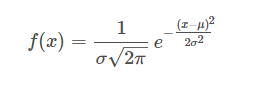公式解释: f(x): 是某样本(样本以数值形式表现)为某数值时发生的概率 0<f(x)<1
x: 是随机抽样的数值,取值范围从负无穷大到正正无穷大 e: 是自然数 σ: 是样本的标准差 μ:是样本的算术平均值(也叫均值)
对服从正太分布下,概率密度函数的理解: (1) 当自变量x=μ时,f(x)取的最大值, 最大值=f(x=μ) 即:当自变量取值为均值时,发生的概率最大(或者说:发生概率最大的数是均值)
(2) 概率密度函数的图像(曲线图像)关于x=μ对称
(3) 标准差σ越大, 则图像峰值(峰值也就是概率最大值,即:峰值=f(x=μ))越小
(4) 概率最小值趋近于0
应用python绘制图像
"""
绘制正太分布函数曲线图

"""
import matplotlib.pyplot as plt
import math
import numpy as np
import matplotlib

matplotlib.rcParams['axes.unicode_minus']=False#解决保存图像时负号'-'显示为方块的问题
plt.rcParams['font.sans-serif'] = ['SimHei'] # 指定默认字体

x = np.linspace(-10,30,num=1000)  # x轴的取值范围
std1 = 1 # 定义标准差, 并输入标准差
mean1 = 10  # 定义均值,并输入均值
fx1 = 1 / (std1 * pow(2 * math.pi, 0.5)) * np.exp(-((x - mean1) ** 2) / (2 * std1 ** 2))  # 概率密度函数公式

std2 = 2
mean2 = 10
fx2 = 1 / (std2 * pow(2 * math.pi, 0.5)) * np.exp(-((x - mean2) ** 2) / (2 * std2 ** 2))  # 概率密度函数公式

std3 = 4
mean3= 10
fx3 = 1 / (std3 * pow(2 * math.pi, 0.5)) * np.exp(-((x - mean3) ** 2) / (2 * std3 ** 2))  # 概率密度函数公式

std4 = 8
mean4 = 10
fx4 = 1 / (std4 * pow(2 * math.pi, 0.5)) * np.exp(-((x - mean4) ** 2) / (2 * std4 ** 2))  # 概率密度函数公式

# 多条曲线在同一张图上进行对比
plt.plot(x, fx1,label = 'std1 = 1')  # 绘制概率密度函数图像
plt.plot(x,fx2,label = 'std2 = 2')
plt.plot(x,fx3,label = 'std3 = 4')
plt.plot(x,fx4,label = 'std4 = 8')
plt.legend() # 显示标签 label
plt.xlabel("数值")
plt.ylabel('数值的概率')
plt.title('服从正太分布的概率密度图')
plt.show()  # 显示图像

显示图像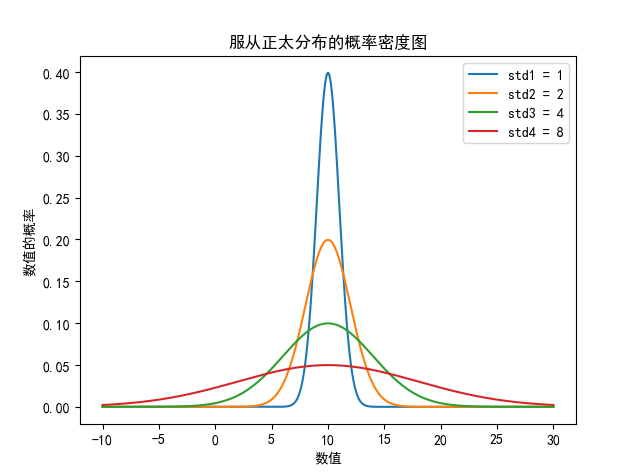由图像可知道: 标准差std 越大, 则峰值越小.(代码设置了均值相同)
稍微修改均值和标准差后,重新绘图, 如下:
import matplotlib.pyplot as plt
import math
import numpy as np
import matplotlib

matplotlib.rcParams['axes.unicode_minus']=False#解决保存图像时负号'-'显示为方块的问题
plt.rcParams['font.sans-serif'] = ['SimHei'] # 指定默认字体

x = np.linspace(-10,30,num=1000)  # x轴的取值范围
std1 = 3 # 定义标准差, 并输入标准差
mean1 = 6  # 定义均值,并输入均值
fx1 = 1 / (std1 * pow(2 * math.pi, 0.5)) * np.exp(-((x - mean1) ** 2) / (2 * std1 ** 2))  # 概率密度函数公式

std2 = 3
mean2 = 10
fx2 = 1 / (std2 * pow(2 * math.pi, 0.5)) * np.exp(-((x - mean2) ** 2) / (2 * std2 ** 2))  # 概率密度函数公式

std3 = 3
mean3= 12
fx3 = 1 / (std3 * pow(2 * math.pi, 0.5)) * np.exp(-((x - mean3) ** 2) / (2 * std3 ** 2))  # 概率密度函数公式

std4 = 3
mean4 = 18
fx4 = 1 / (std4 * pow(2 * math.pi, 0.5)) * np.exp(-((x - mean4) ** 2) / (2 * std4 ** 2))  # 概率密度函数公式

# 多条曲线在同一张图上进行对比
plt.plot(x, fx1,label = '均值 = 6')  # 绘制概率密度函数图像
plt.plot(x,fx2,label = '均值 = 10')
plt.plot(x,fx3,label = '均值 = 12')
plt.plot(x,fx4,label = '均值 = 18')
plt.legend() # 显示标签 label
plt.xlabel("数值")
plt.ylabel('数值的概率')
plt.title('服从正太分布的概率密度图')
plt.show()  # 显示图像

图像显示: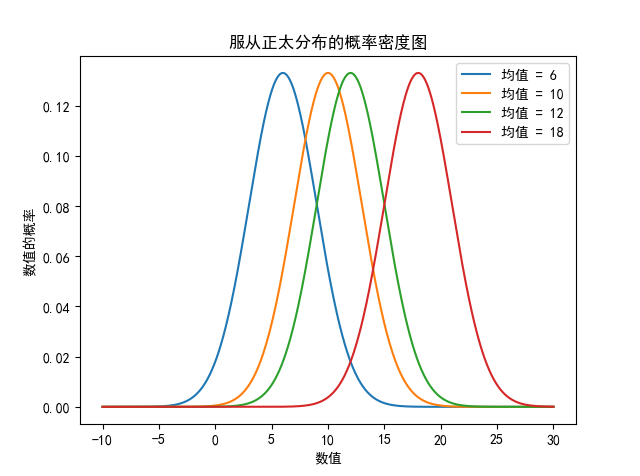由图像看出, 当标准差相同时(代码设置标准差相同), 峰值相同, 均值不同时, 对称轴位置不同
再次修改代码对标准差和均值重新赋值(可跳过不用看代码, 直接看图像)
import matplotlib.pyplot as plt
import math
import numpy as np
import matplotlib

matplotlib.rcParams['axes.unicode_minus']=False#解决保存图像时负号'-'显示为方块的问题
plt.rcParams['font.sans-serif'] = ['SimHei'] # 指定默认字体

x = np.linspace(-10,30,num=1000)  # x轴的取值范围
std1 = 3 # 定义标准差, 并输入标准差
mean1 = 6  # 定义均值,并输入均值
fx1 = 1 / (std1 * pow(2 * math.pi, 0.5)) * np.exp(-((x - mean1) ** 2) / (2 * std1 ** 2))  # 概率密度函数公式

std2 = 5
mean2 = 10
fx2 = 1 / (std2 * pow(2 * math.pi, 0.5)) * np.exp(-((x - mean2) ** 2) / (2 * std2 ** 2))  # 概率密度函数公式

std3 = 6
mean3= 12
fx3 = 1 / (std3 * pow(2 * math.pi, 0.5)) * np.exp(-((x - mean3) ** 2) / (2 * std3 ** 2))  # 概率密度函数公式

std4 = 2
mean4 = 18
fx4 = 1 / (std4 * pow(2 * math.pi, 0.5)) * np.exp(-((x - mean4) ** 2) / (2 * std4 ** 2))  # 概率密度函数公式

# 多条曲线在同一张图上进行对比
plt.plot(x, fx1,label = '均值 = 6, 标准差=3')  # 绘制概率密度函数图像
plt.plot(x,fx2,label = '均值 = 10, 标准差=5')
plt.plot(x,fx3,label = '均值 = 12, 标准差=6')
plt.plot(x,fx4,label = '均值 = 18, 标准差=2')
plt.legend() # 显示标签 label
plt.xlabel("数值")
plt.ylabel('数值的概率')
plt.title('服从正太分布的概率密度图')
plt.show()  # 显示图像

图像显示: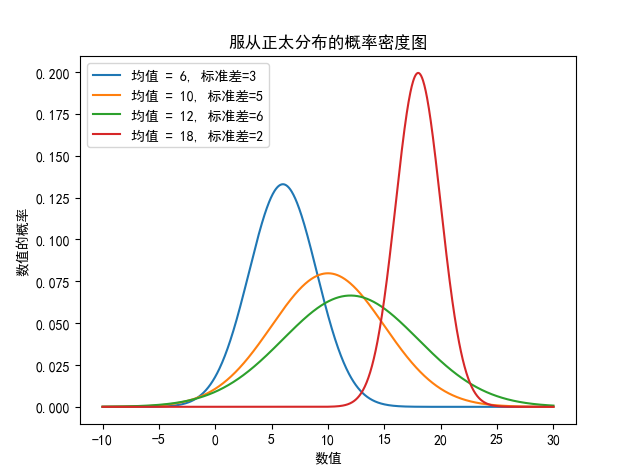通过图像可以观查出均值和标准差对概率的影响, 验证了上述的结论
最后: 本人又另写一篇关于服从正太分布概率 离散情况下 概率密度函数和累积密度函数的文章. 有疑惑的可以查看一下
展开全文正态分布
• mumpy 、random import numpy as np import seaborn as sns import matplotlib.pyplot as plt from scipy import stats np.random.seed(1234) rn1 = np.random.normal(loc = 0, scale = 1, size = 1000) ...
mumpy 、random
import numpy as np
import seaborn as sns
import matplotlib.pyplot as plt
from scipy import stats

np.random.seed(1234)
rn1 = np.random.normal(loc = 0, scale = 1, size = 1000)
rn2 = np.random.normal(loc = 0, scale = 2, size = 1000)
rn3 = np.random.normal(loc = 2, scale = 3, size = 1000)
rn4 = np.random.normal(loc = 5, scale = 3, size = 1000)

#绘图
plt.style.use('ggplot')
sns.distplot(rn1, hist=False, kde=False, fit=stats.norm, fit_kws={'color':'black','label':'u=0,s=1','linestyle':'-'})
sns.distplot(rn2, hist=False, kde=False, fit=stats.norm, fit_kws={'color':'red','label':'u=0,s=2','linestyle':'--'})
sns.distplot(rn3, hist=False, kde=False, fit=stats.norm, fit_kws={'color':'blue','label':'u=2,s=3','linestyle':':'})
sns.distplot(rn4, hist=False, kde=False, fit=stats.norm, fit_kws={'color':'purple','label':'u=5,s=3','linestyle':'-.'})

#呈现图例
plt.legend()
#呈现图形
plt.show()展开全文• 文章目录术语前言整数浮点数抽取字节洗牌排列贝塔分布二项分布卡方分布狄利克雷分布指数分布F分布伽玛分布几何分布耿贝尔分布超几何分布拉普拉斯分布（双指数分布）逻辑斯谛分布正态...pdf，概率密度函数（Probability
• 使用numpy和matplotlib.pyplot绘制正态分布、两点分布、二项分布 、几何分布、均匀分布 、指数分布曲线
推荐阅读：如何理解概率分布函数和概率密度函数

文章目录
正态分布(Nomal Distribution)两点分布 /伯努利分布(Two Point Distribution)二项分布 (Binomial Distribution)几何分布 (Geometric Distribution)泊松分布（Possion Distribution)均匀分布 (Uniform Distribution)指数分布 (Exponential Distribution)

正态分布(Nomal Distribution)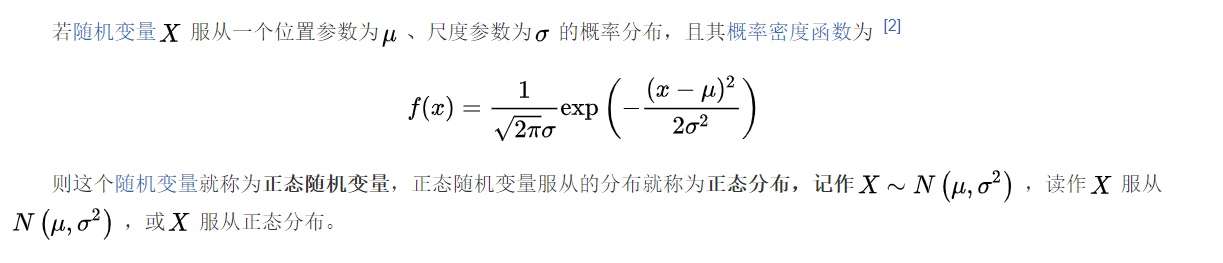import numpy as np
import matplotlib.pyplot as plt
import math
# 均值
u = 0
#标准差
sig = math.sqrt(0.2)

x = np.linspace(u - 3 * sig, u + 3 * sig, 50)
y_sig = np.exp(-(x - u) ** 2 / (2 * sig ** 2) / (math.sqrt(2 * math.pi) * sig))
plt.plot(x,y_sig,"r-",linewidth=2)
plt.grid(True)
plt.show()

正态分布概率密度函数曲线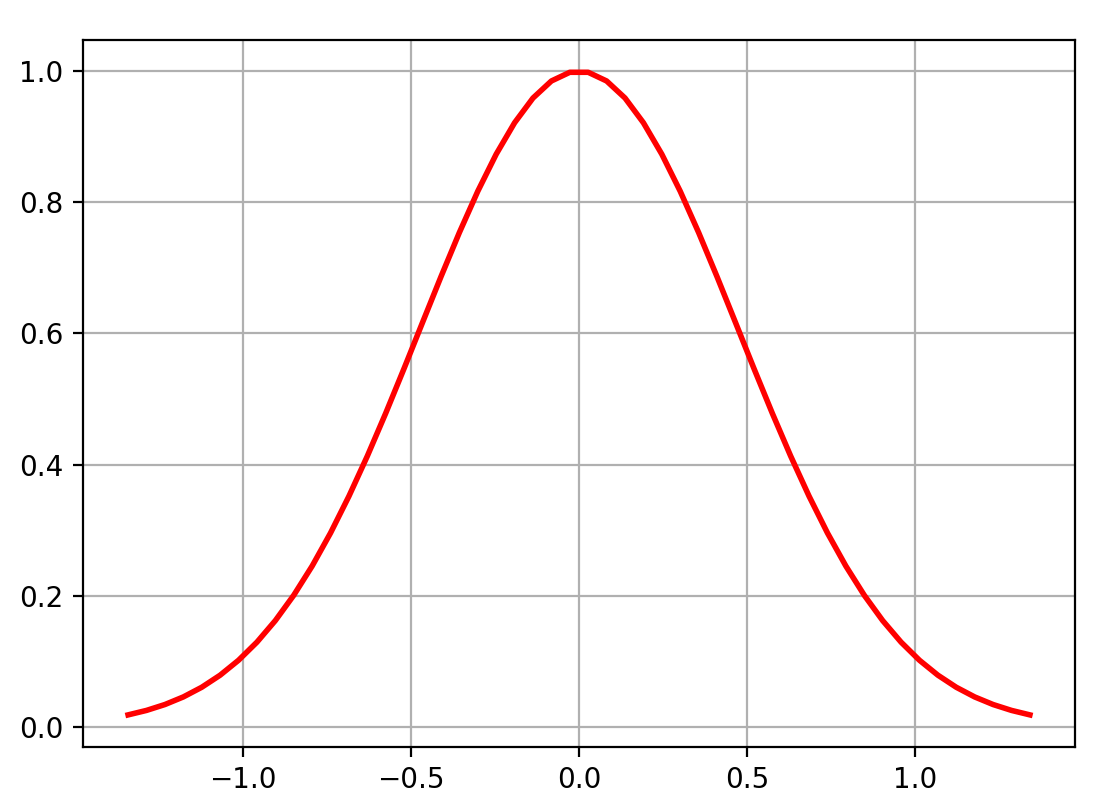两点分布 /伯努利分布(Two Point Distribution)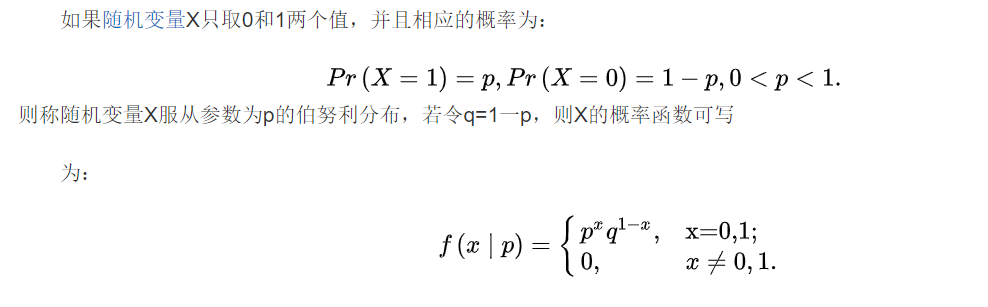import numpy as np
import matplotlib.pyplot as plt
p = 0.7
x = [0,1]
y = [1-p, p]
plt.scatter(x,y) # 散点图
plt.grid(True)
plt.show()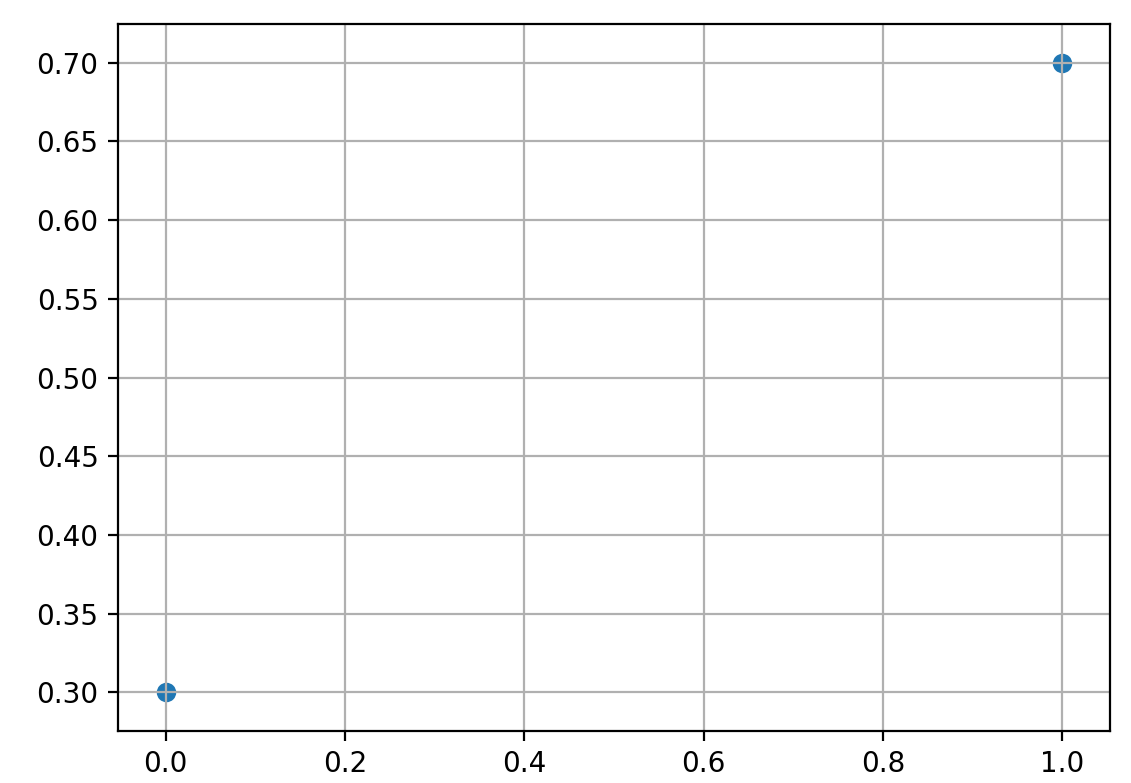二项分布 (Binomial Distribution)
二项分布，即重复n次的伯努利试验，用ξ表示随机试验的结果。如果事件发生的概率是p,则不发生的概率q=1-p，N次独立重复试验中发生K次的概率是 P(ξ=K)= C(n,k) * p^k * (1-p)^(n-k)，其中C(n, k) =n!/(k!(n-k)!). 那么就说这个属于二项分布。其中P称为成功概率。记作ξ~B(n,p) 期望：Eξ=np； 方差：Dξ=npq, 其中q=1-p
import numpy as np
import matplotlib.pyplot as plt
from scipy.special import comb
p = 0.4
n = 10
x = np.linspace(0,n,n+1)
y = comb(n,x)*p**x*(1-p)**(n-x)
print(x)
print(y)
plt.scatter(x,y)
plt.grid(True)
plt.show()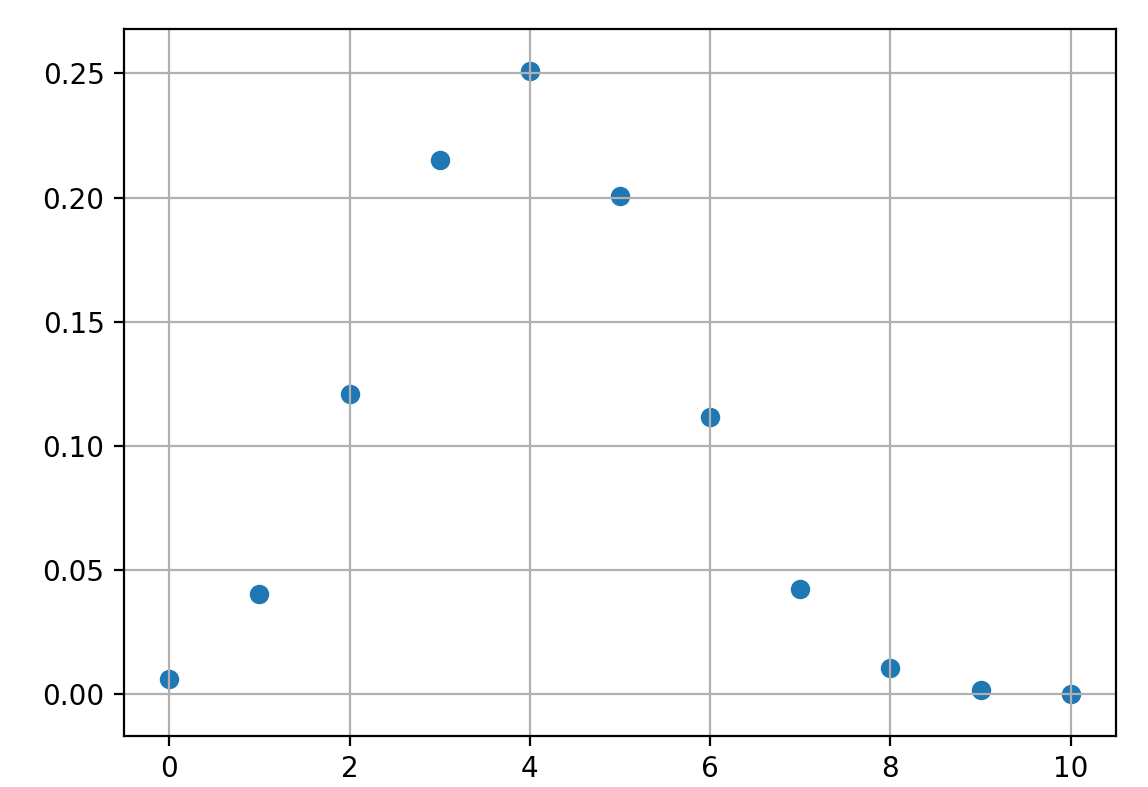几何分布 (Geometric Distribution)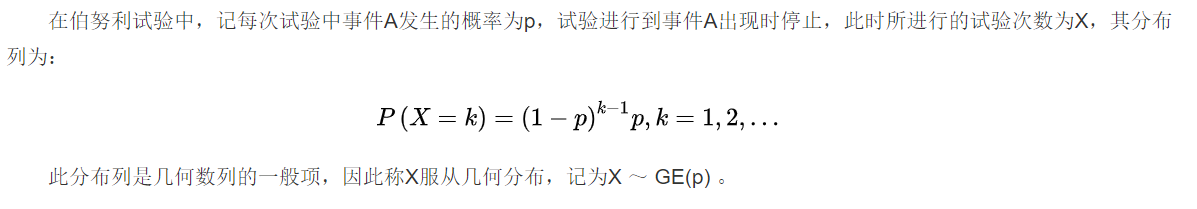import numpy as np
import matplotlib.pyplot as plt
p = 0.4
n = 10
x = np.linspace(1,n,n)
y = p*(1-p)**(x-1)
print(x)
print(y)
plt.scatter(x,y)
plt.grid(True)
plt.show()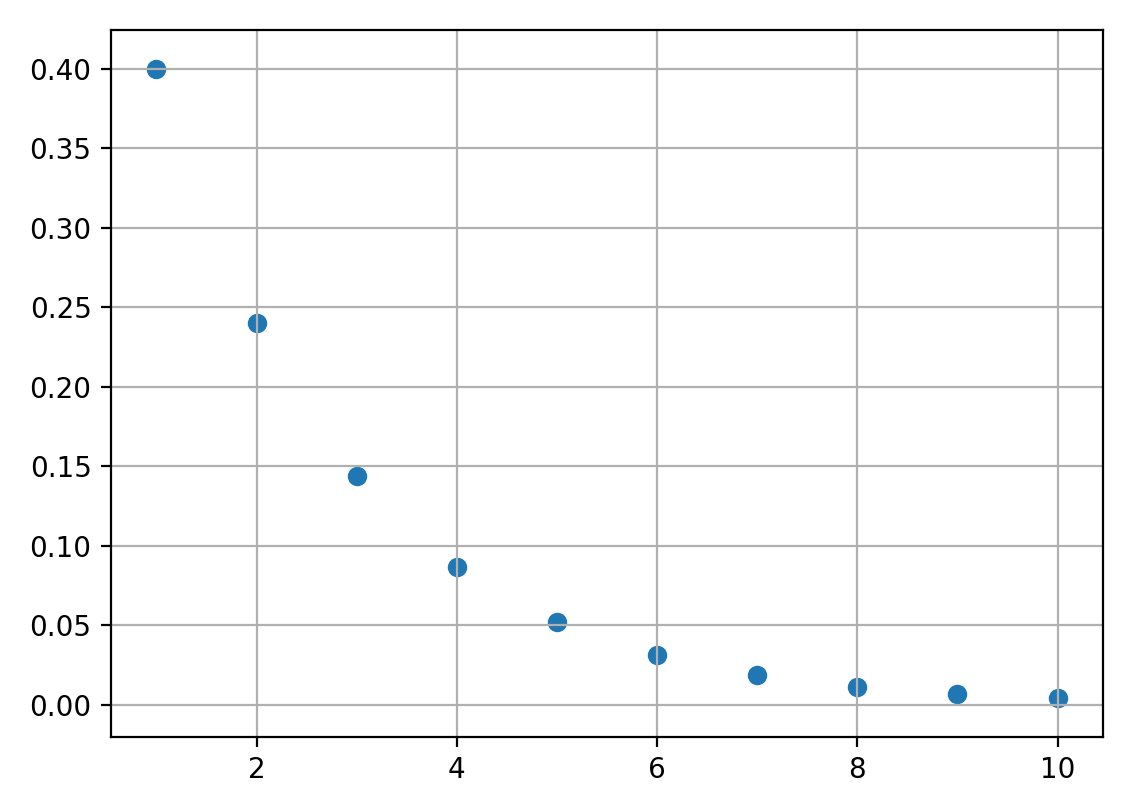泊松分布（Possion Distribution)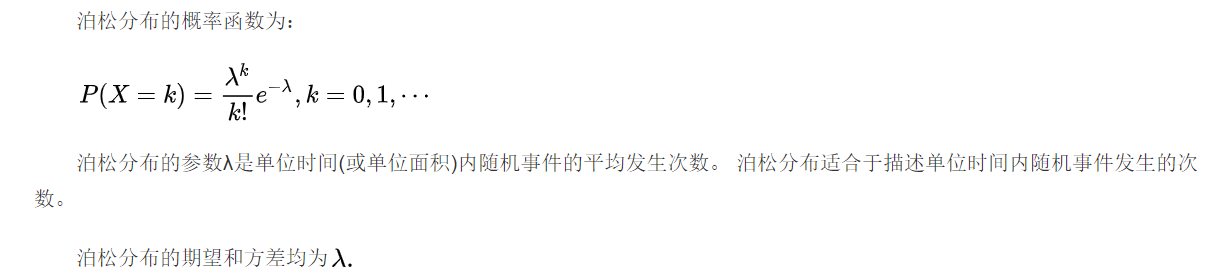import numpy as np
import matplotlib.pyplot as plt

x = np.random.poisson(lam = 5, size = 10000)
pillar = 15
a = plt.hist(x, pillar, color = 'g')
plt.plot(a[0:pillar], a,'r')
plt.grid()
plt.show()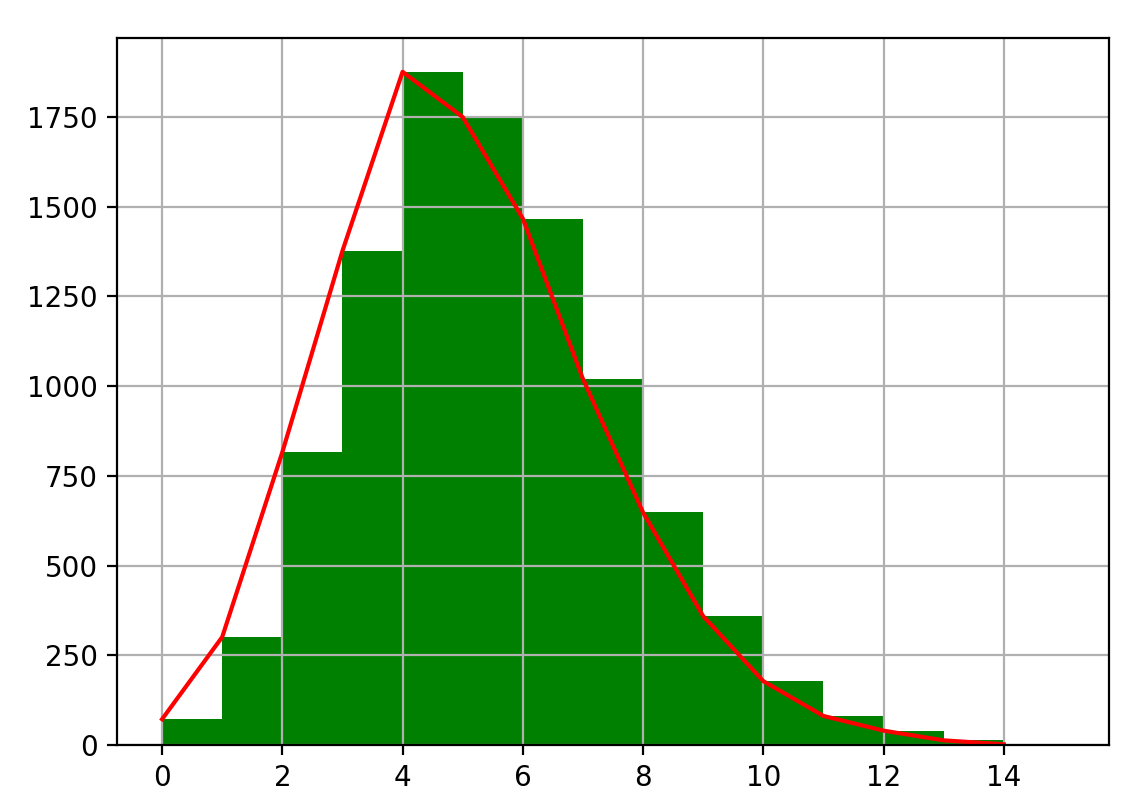均匀分布 (Uniform Distribution)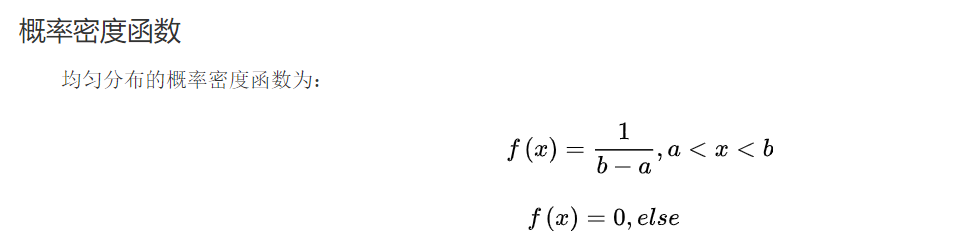import numpy as np
import matplotlib.pyplot as plt
a = 3
b = 5
x = np.linspace(a, b, 50)
y = []
for i in range(0,50):
y.append(1 / (b - a))
plt.plot(x,y,"r-",linewidth=2)
plt.grid(True)
plt.show()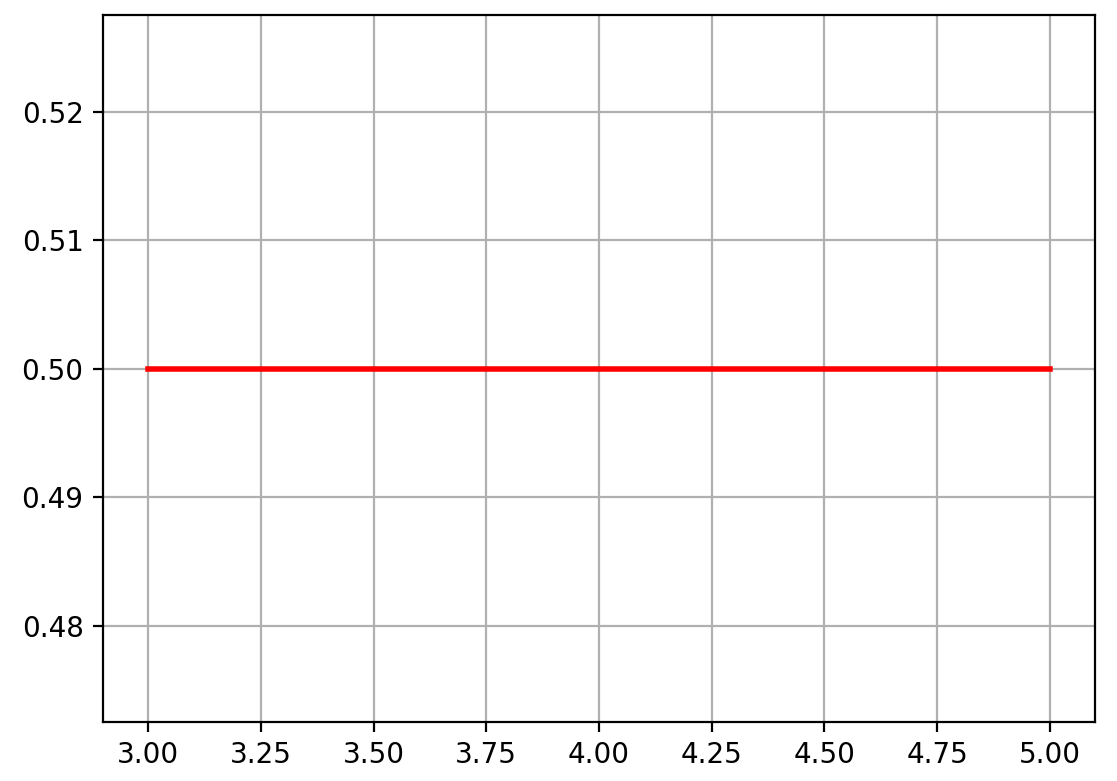指数分布 (Exponential Distribution)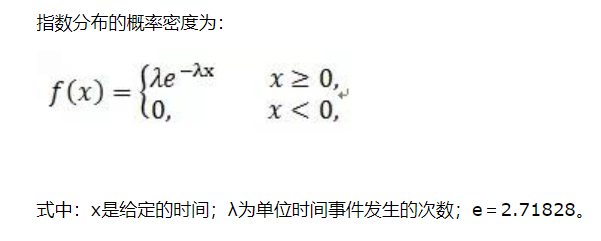import numpy as np
import matplotlib.pyplot as plt

x = np.random.exponential(scale = 100, size = 10000)
pillar = 25
a = plt.hist(x, pillar, color = 'g')
plt.plot(a[0:pillar], a,'r')
plt.grid()
plt.show()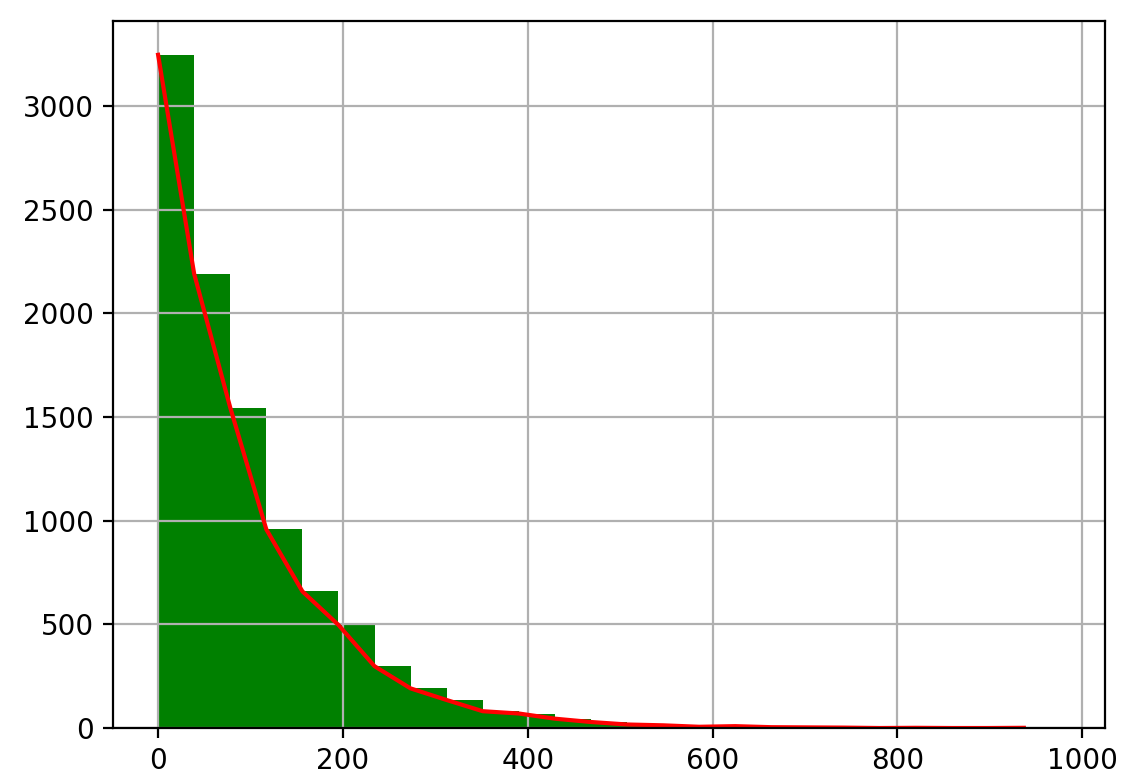展开全文统计学
• 二维高斯分布概率密度函数数据集实战优化坐标轴与图像优化图像再次优化 概率密度函数 大家肯定都有听说过正态分布，其实正态分布只是概率密度分布的一种，正态分布的概率密度函数均值为μ ，标准差σ是高斯函数的一...


二维高斯分布
概率密度函数数据集实战优化坐标轴与图像优化图像再次优化

概率密度函数
大家肯定都有听说过正态分布，其实正态分布只是概率密度分布的一种，正态分布的概率密度函数均值为μ ，标准差σ是高斯函数的一个实例：

f

(

x

;

μ

,

σ

)

=

1

σ

2

π

exp

⁡

(

−

(

x

−

μ

)

2

2

σ

2

)

f(x ; \mu, \sigma)=\frac{1}{\sigma \sqrt{2 \pi}} \exp \left(-\frac{(x-\mu)^{2}}{2 \sigma^{2}}\right)

在一维上只有x一个变量，μ 均值，σ标准差。 正态分布具有两个参数μ和σ的连续型随机变量的分布，第一 参数μ是服从正态分布的随机变量的均值，第二个参数σ^2是此随机变量的方差，所以正态分布记作N（μ,σ2）。
实际工作中，正态曲线下横轴上一定区间的面积反映该区间的例数占总例数的百分比，或变量值落在该区间的概率。
因此一维的概率密度分布即正态分布，很好的表示数据在哪个区间集中，使我们对整体数据有一个大概的把握。
本文的重点在于二维概率密度函数：

f

(

x

,

y

)

=

(

2

π

σ

1

σ

2

1

−

ρ

2

)

−

1

exp

⁡

[

−

1

2

(

1

−

ρ

2

)

(

(

x

−

μ

1

)

2

σ

1

2

−

2

ρ

(

x

−

μ

1

)

(

y

−

μ

2

)

σ

1

σ

2

+

(

y

−

μ

2

)

2

σ

2

2

)

]

f(x, y)=\left(2 \pi \sigma_{1} \sigma_{2} \sqrt{1-\rho^{2}}\right)^{-1} \exp \left[-\frac{1}{2\left(1-\rho^{2}\right)}\left(\frac{\left(x-\mu_{1}\right)^{2}}{\sigma_{1}^{2}}-\frac{2 \rho\left(x-\mu_{1}\right)\left(y-\mu_{2}\right)}{\sigma_{1} \sigma_{2}}+\frac{\left(y-\mu_{2}\right)^{2}}{\sigma_{2}^{2}}\right)\right]

因为生活中的很多数据都是高维度的，从简单的二维说起。二维上的数据生活中有很多：身高和体重，血压和血脂等等。如果能够像一维正态分布那样做出图像来看，就十分直观，而本文就是介绍如何作二维概率密度函数的图像。
数据集
首先贴上数据集： 链接：https://pan.baidu.com/s/1RJCwi4-8_hByY6-rCepJgQ 提取码：88ew
数据是截至4.25日的重点国家新冠肺炎感染人数，有中国、美国、法国、意大利等。 本文采取的是中国和意大利进行对比分析。
import numpy as np
import matplotlib.pyplot as plt
import math
import mpl_toolkits.mplot3d
import math
import pandas as pd

x = data.iloc[:,1]
y = data.iloc[:,7]
x = x.values
y = y.values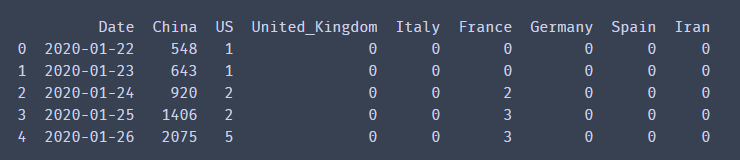实战
首先根据公式我们先把2个维度的均值和方差分别计算出来，以及公式中需要的相关系数。

u1 = x.mean()
u2 = y.mean()
o1 = x.std()
o2 = y.std()
from scipy.stats import pearsonr
p = pearsonr(x, y)
print(u1, u2, o1, o2, p)

# 输出:(r, p)
# r:相关系数[-1，1]之间
# p:相关系数显著性

相关系数也就是皮尔逊系数，把2个维度数据给入后，会输出相关系数和相关系数显著性。 相关系数取值范围是(-1，1)，越接近1则说明越相关。不过我们也不能说中国感染人数和西班牙感染人数相关，这里更确切地解释应该是感染人数的趋势比较。
X, Y = np.meshgrid(x, y)
z = (1/(2*math.pi*o1*o2*pow(1-pow(p,2),0.5)))*np.exp(-1/(2*(1-p*p))*(((X-u1)*(X-u1))/(o1*o1)-2*p*(X-u1)*(Y-u2)/(o1*o2)+(Y-u2)*(Y-u2)/(o2*o2)))

这里X,Y是对原始数据进行网格化，其实就相当于最后成果图的横纵坐标，只是转换一下得以输入作图。 z就是上文的二维密度函数用python来表达了。比较麻烦，注意里面有上面算出的2个维度的均值，方差和皮尔逊系数。
plt.rcParams['font.sans-serif'] = ['KaiTi']  # 用来正常显示中文字符
plt.rcParams['axes.unicode_minus'] = False
plt.figure(figsize=(10,10), dpi=300)
ax = plt.subplot(111, projection='3d')
ax.plot_surface(X, Y, z,
cmap='rainbow', alpha=0.9)
ax.set_xlabel('中国感染人数')
ax.set_ylabel('西班牙感染人数')
ax.set_zlabel('频率')
ax.set_title("二维高斯分布")

这就是很基础的一些画图设置了，相似的就不再赘述，重点 谈谈plot_surface。 plot_surface中的X，Y，z其实上文以及解释过了，就是相应的坐标和函数，那么cmap是什么呢，camp是颜色盘，值定位rainbow就是彩虹色，从下图就可以看出，数据越集中的地方，颜色就越深。这里还有一个颜色盘是coolwarm，不过个人感觉没rainbow好看，不妨小伙伴们试一试。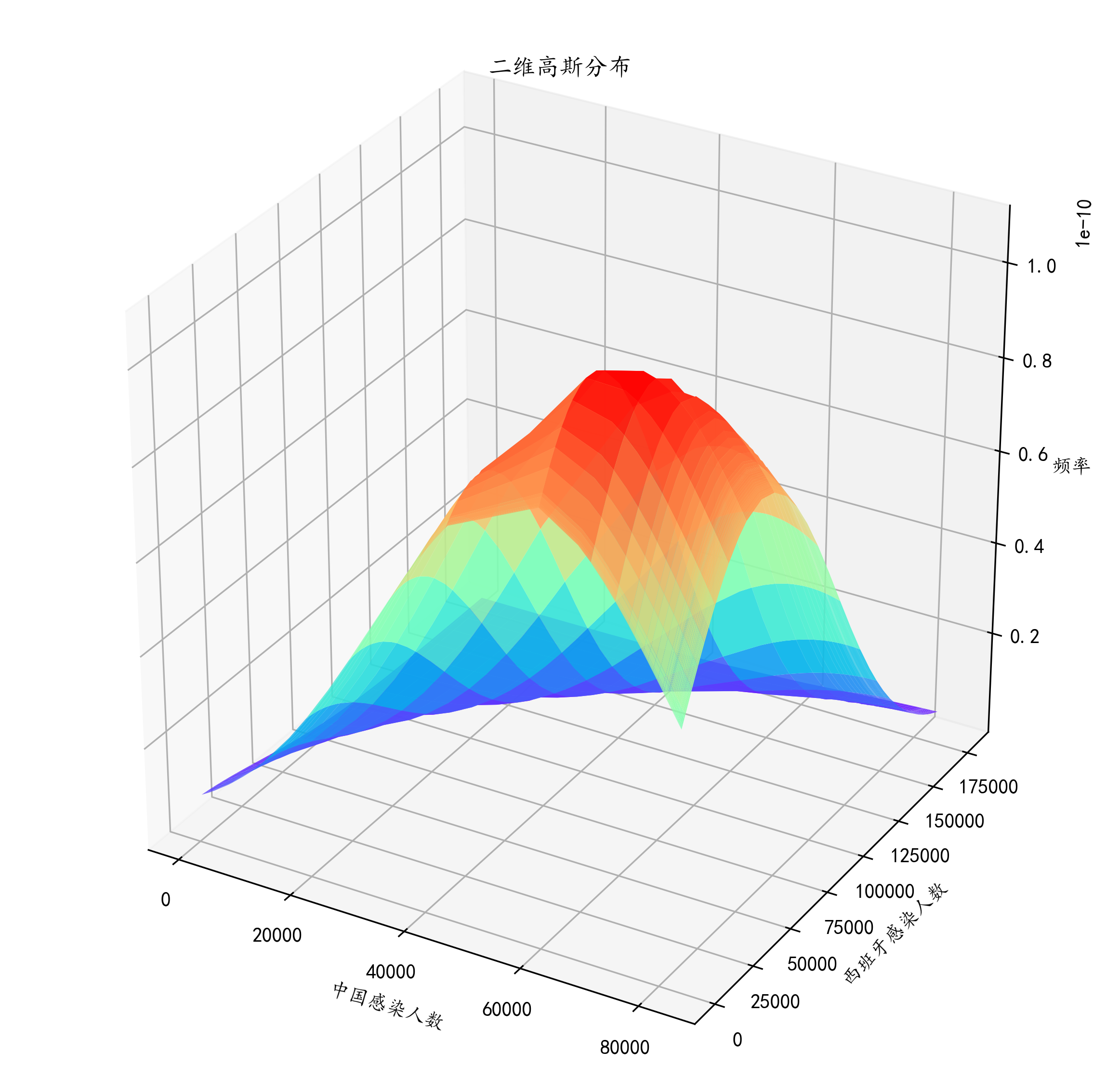到此我们就大概的画出了中国感染人数和西班牙感染人数在4.25之前的密度函数。 这个图我们看出，中国感染人数大概在4-5万就开始达到高峰，之后开始下降，而西班牙到了12万左右才开始下降。整个国家感染人数的增幅一目了然，对于整体数据的把握也有较好的认知。但这样似乎不太好看，而且到底高峰是不是在我说的那个数值呢，根据肉眼都不好判断。所以我们接下来进行优化。
优化
坐标轴与图像优化
plt.rcParams['font.sans-serif'] = ['KaiTi']  # 用来正常显示中文字符
plt.rcParams['axes.unicode_minus'] = False
plt.figure(figsize=(10,10), dpi=300)
ax = plt.subplot(111, projection='3d')
ax.plot_surface(X, Y, z, rstride=1, cstride=1,
cmap='rainbow', alpha=0.9)
ax.set_xlabel('中国感染人数', fontsize=15)
ax.set_ylabel('西班牙感染人数', fontsize=15)
ax.set_zlabel('频率', fontsize=15)
ax.set_title("二维高斯分布", fontsize=25, y=1.02)

ax.set_xticks(np.arange(0,100000,20000))
ax.set_yticks(np.arange(0,200000,40000))

可能一眼还没看出来。我来讲解一下。博主在plot_surface里面加了 rstride=1, cstride=1,这两个参数有什么作用？相当于步长。这么理解吧，这个颜色实际上是由无数个点组成的，但是实际上就像房子顶上的瓦片一样，如果瓦片比较大，那么房顶面积一定，瓦片就用的少，就像上图一样显得一块一块的，非常大，不过不平滑。而下图呢，加入 rstride=1, cstride=1就相当于定制了瓦片长宽，瓦片比较小那么看起来就舒服，颜色过渡得比上面那个自然多。 把标题和坐标轴都修改一下，title的x，y参数是调位置的，如何使用的话小伙伴们多试几个值就明白了。 plt.savefig里面的bbox_inches=‘tight’, pad_inches=0.0在这里看起里效果似乎不明显。这个作用是减小图片旁边的白色区域。如果感兴趣的小伙伴可以试一下不加和加了这些参数保存出来是什么样的。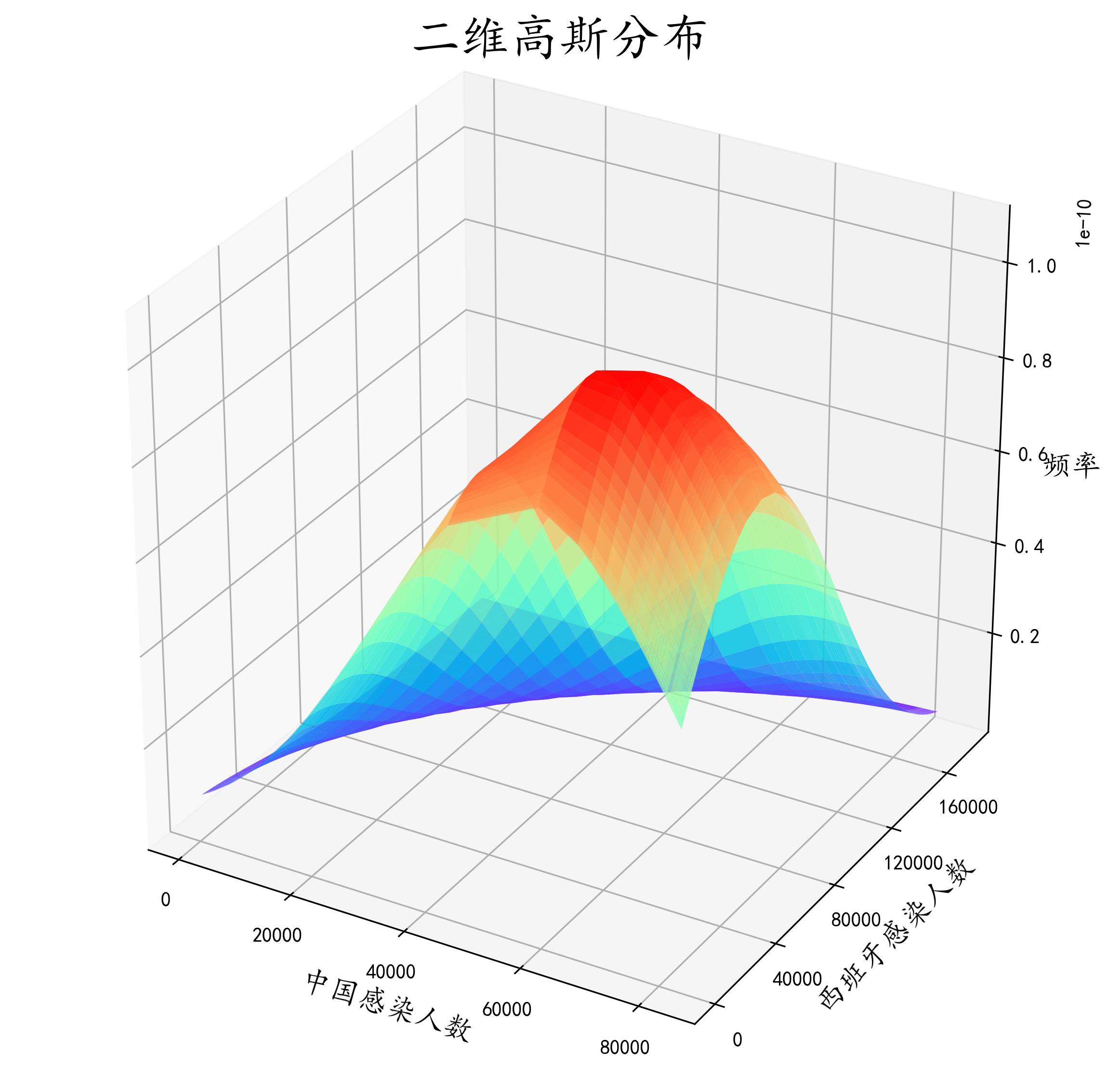图像再次优化
plt.rcParams['font.sans-serif'] = ['KaiTi']  # 用来正常显示中文字符
plt.rcParams['axes.unicode_minus'] = False
plt.figure(figsize=(10,10), dpi=300)
ax = plt.subplot(111, projection='3d')
ax.plot_surface(X, Y, z, rstride=1, cstride=1,
cmap='rainbow', alpha=0.9)
ax.set_xlabel('中国感染人数', fontsize=15)
ax.set_ylabel('西班牙感染人数', fontsize=15)
ax.set_zlabel('频率', fontsize=15)
ax.set_title("二维高斯分布", fontsize=25, y=1.02)

ax.set_xticks(np.arange(0,100000,20000))
ax.set_yticks(np.arange(0,200000,40000))
ax.contour(X, Y, z, 15, zdir = 'z', offset = 0, cmap = plt.get_cmap('rainbow'))
ax.w_xaxis.set_pane_color((135/255, 206/255, 250/255, 0.3))
ax.w_yaxis.set_pane_color((135/255, 206/255, 250/255, 0.3))
ax.w_zaxis.set_pane_color((135/255, 206/255, 250/255, 0.3))


作图一方面为了好看，一方面是对数据整体把握更加直观，这里加了ax.w_xaxis.set_pane_color这个方法是对x平面进行上色，个人感觉更好看吧。里面的参数是rgba。 细心的小伙伴已经发现了，这个图比上面的多了好多等高线。这些等高线是这个密度函数在xoy平面的投影，能够更直观的看出到底数据的高峰是在哪。我们直观看出，中国感染人数到达高峰是在6w人左右，而西班牙也是在6w人左右，这和我们前面目测估计的有一点误差。所以ax.contour这个方法将密度函数投影到平面来，更细致的观察数据的分布。其中ax.contour中 15代表是有多少条等高线，zdir=z表示投影到z=？这个平面，而？的数值就是由offset表示，这里显然投影到z=0平面，camp也是和上文意思差不多是颜色盘，彩虹色的。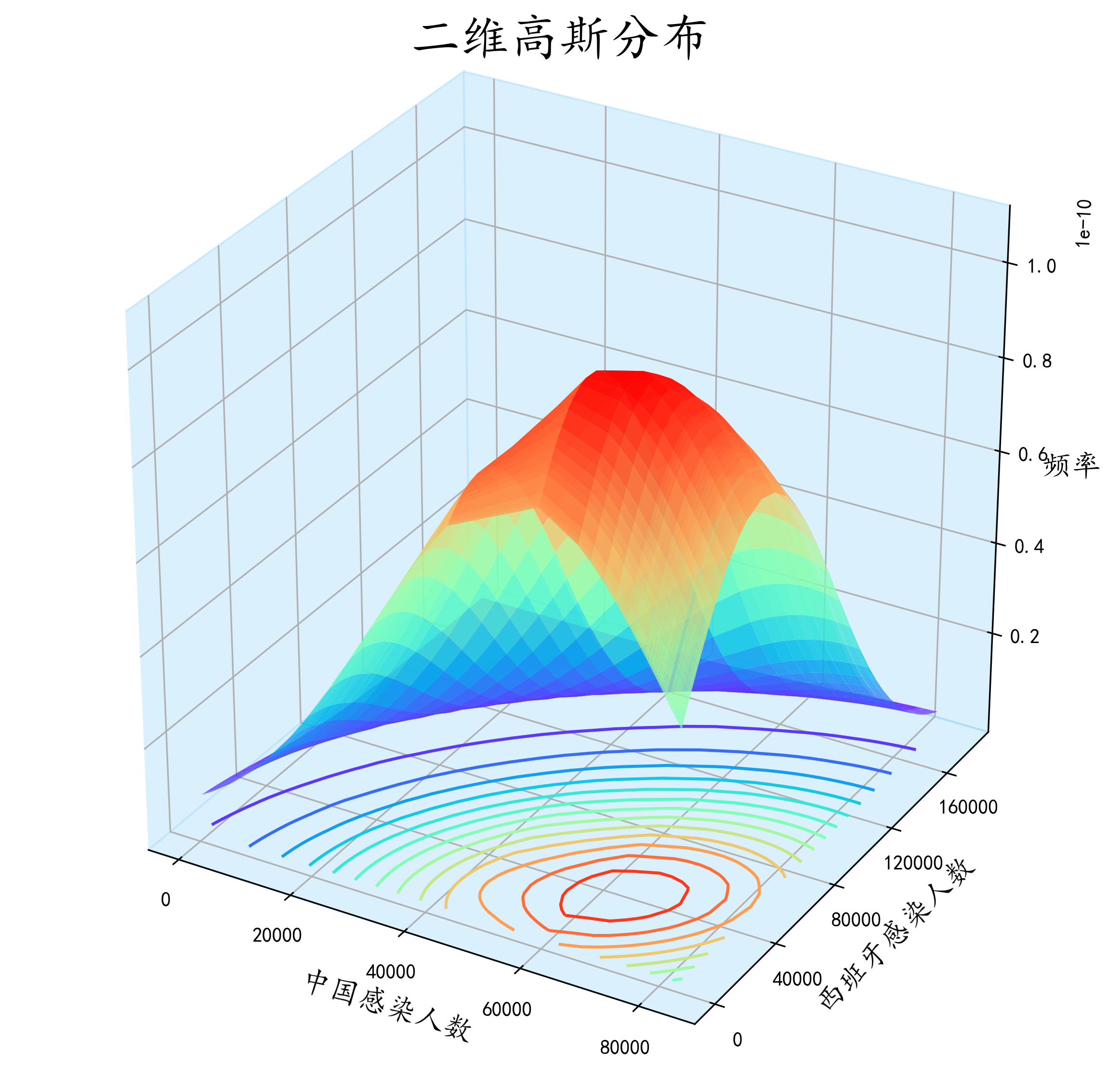效果还是很直观的。
展开全文数据分析 机器学习
• python 服从正太分布 离散情况 下 的概率密度函数 scipy.stats库是分布函数库, 包含正太分布,泊松分布,卡方分布等 导入scipy.stats库以后, 应用函数norm生成服从正太分布的概率密度函数, 具体法是: scipy...norm
• 今天使用python画了几个好玩的3D展示图，现在分享给大家。 先贴上图片 ...在第三张图片中，我们可以理解为，z是随着x和y变化的函数。就像一个人在山丘地区走动一样，其中x和y表示的是方向，z表示的这个
• miu = 0 sigma = 1 x = np.linspace(miu - 3 * sigma, miu + 3 * sigma, 50) y = np.exp(-(x - miu) ** 2 / (2 * sigma ** 2)...plt.title('高斯分布函数', fontsize=18) plt.grid(True) plt.show()标准正态分布 matplotlib
• 利用input()函数输入均值和标准差, 多次绘制概率密度函数图形并将图像曲线放置在同一张图中 代码块: """ 绘制正太分布函数曲线图 """ import matplotlib.pyplot as plt import math import numpy as np import ...循环输入 正太分布
• 概率密度函数（pdf）针对的是连续变量，例如正态分布 而概率质量函数（pmf）针对的是随机变量，例如泊松分布。 之前一直没有注意到这个差别，貌似大学的概率课本上将二者等同了。...概率质量函数 pmf
• 主要介绍了Python绘制的二项分布概率图,涉及Python基于numpy、math的数值运算及matplotlib图形绘制相关操作技巧,需要的朋友可以参考下
• 计算分布函数(也称累积概率函数CDF) python代码如下： import numpy as np data = np.random.randn(200) # 随机生成一些数 # 一个公式即可计算出概率函数 cdf_value = 1. * np.arange(len(data)) / (len(data) - 1...
• import seaborn as sns plt.figure(figsize=(6, 4)) sns.kdeplot(data, shade=True, color="g", alpha=.7) plt.xlabel('xlabel') plt.tight_layout() plt.savefig(path)
• plt.plot(np.linspace(-3,3,100),stats.norm.pdf(np.linspace(-3,3,100))) #从（-3，3）中随机选取100个数，绘制该事件的概率密度函数 plt.fill_between(np.linspace(-3,3,100),stats.norm.pdf(np.linspace(-3,3,100...统计学
• 鉴于单峰的状态分布公式是： f(x)=(1/((2π)^0.5)*σ)exp-((x-a)^2/(2*σ^2)) 于是N个平均值。...这里每个i对应一个独立的平均值ai、标准差σi,以及它的概率峰位置，而各个ki是一个系数，并且它们...
• 简单对概率分布函数进行一个描述，在概率论中要研究一个随机变量ξ取值小于某一数值x的概率，这概率是x的函数，称这种函数为随机变量ξ的分布函数。 F(x)=P(X<x),F(+∞)=1,F(−∞)=0F(x)=P(X<x),F(+\infin)=1,...
• Matplotlib在概率密度曲线图的基础上绘制积分区域，并撰写积分表达式。
• python实现描述性统计、频数分布图、正态分布检验、概率密度曲线拟合 from scipy.stats import chi2 # 卡方分布 from scipy.stats import norm # 正态分布 from scipy.stats import t # t分布 from scip...数据分析 正态分布检验
• 对于pandas的dataframe，绘制直方图方法如下： //pdf是pandas的dataframe, delta_time...对于pandas的dataframe，绘制密度图方法如下： //pdf是pandas的dataframe, delta_time是其中一列 pdf.delta_time.dropna()...
• 累积分布函数(Cumulative Distribution Function)，又叫分布函数，是概率密度函数的积分，能完整描述一个实随机变量X的概率分布。在电子器件制造中常常用于描述器件失效分布，可以明显的表示出在特定数值下的器件...数据分析 可视化
• 我们在统计数据处理时，经常计算一个样本的概率密度估计，也就是说给出一组统计数据，要求你绘制出它的概率分布曲线，matlab的统计工具箱中有直接的函数就是：Ksdensity核心平滑密度估计 [f,xi] = ksdensity(x) ...
• import numpy.random as npr import matplotlib.pyplot as plt from pylab import mpl mpl.rcParams['font.sans-serif'] = ['SimHei'] mpl.rcParams['axes.unicode_minus'] = False I=1000 x_norm = npr.normal(l...
• python绘制累积正态分布概率图 累积正态分布概率图，即正态分布函数的积分形式。其公式为： 去掉积分符号就是正态分布的公式。 以下是实现的代码。 // A code block import numpy as np import matplotlib.pyplot ...数据分析...

# python绘制概率密度函数python 订阅